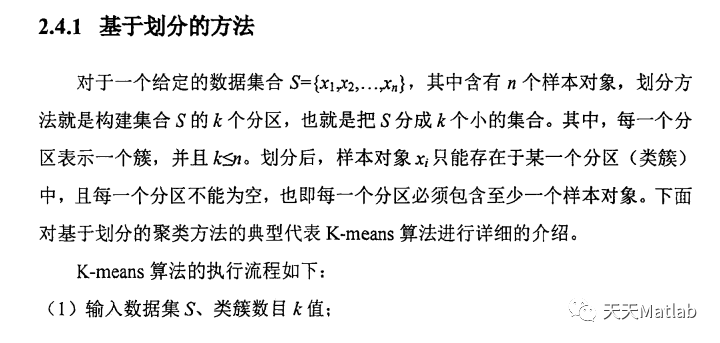# 【原创】数据挖掘案例——ReliefF和K-means算法的医学应用

+关注继续查看

数据挖掘方法的提出，让人们有能力最终认识数据的真正价值，即蕴藏在数据中的信息和知识。数据挖掘 (DataMiriing)，指的是从大型数据库或数据仓库中提取人们感兴趣的知识，这些知识是隐含的、事先未知的潜在有用信息，数据挖掘是目前国际上，数据库和信息决策领域的最前沿研究方向之一。因此分享一下很久以前做的一个小研究成果。也算是一个简单的数据挖掘处理的例子。

# 1.数据挖掘与聚类分析概述

(l)分析问题:源数据数据库必须经过评估确认其是否符合数据挖掘标准。以决定预期结果，也就选择了这项工作的最优算法。

(2)提取、清洗和校验数据:提取的数据放在一个结构上与数据模型兼容的数据库中。以统一的格式清洗那些不一致、不兼容的数据。一旦提取和清理数据后，浏览所创建的模型，以确保所有的数据都已经存在并且完整。

(3)创建和调试模型:将算法应用于模型后产生一个结构。浏览所产生的结构中数据，确认它对于源数据中“事实”的准确代表性，这是很重要的一点。虽然可能无法对每一个细节做到这一点，但是通过查看生成的模型，就可能发现重要的特征。

(4)查询数据挖掘模型的数据:一旦建立模型，该数据就可用于决策支持了。

(5)维护数据挖掘模型:数据模型建立好后，初始数据的特征，如有效性，可能发生改变。一些信息的改变会对精度产生很大的影响，因为它的变化影响作为基础的原始模型的性质。因而，维护数据挖掘模型是非常重要的环节。

聚类分析是数据挖掘采用的核心技术，成为该研究领域中一个非常活跃的研究课题。聚类分析基于”物以类聚”的朴素思想，根据事物的特征，对其进行聚类或分类。作为数据挖掘的一个重要研究方向，聚类分析越来越得到人们的关注。聚类的输入是一组没有类别标注的数据，事先可以知道这些数据聚成几簇爪也可以不知道聚成几簇。通过分析这些数据，根据一定的聚类准则，合理划分记录集合，从而使相似的记录被划分到同一个簇中，不相似的数据划分到不同的簇中。

# 2.特征选择与聚类分析算法

Relief为一系列算法，它包括最早提出的Relief以及后来拓展的ReliefFRReliefF，其中RReliefF算法是针对目标属性为连续值的回归问题提出的，下面仅介绍一下针对分类问题的ReliefReliefF算法。

## 2.1 Relief算法

Relief算法最早由Kira提出，最初局限于两类数据的分类问题Relief算法是一种特征权重算法(Feature weighting algorithms)，根据各个特征和类别的相关性赋予特征不同的权重，权重小于某个阈值的特征将被移除。Relief算法中特征和类别的相关性是基于特征对近距离样本的区分能力。算法从训练集D中随机选择一个样本R，然后从和R同类的样本中寻找最近邻样本H，称为Near Hit，从和R不同类的样本中寻找最近邻样本M，称为NearMiss，然后根据以下规则更新每个特征的权重：如果RNear Hit在某个特征上的距离小于RNear Miss上的距离，则说明该特征对区分同类和不同类的最近邻是有益的，则增加该特征的权重；反之，如果RNear Hit在某个特征的距离大于RNear Miss上的距离，说明该特征对区分同类和不同类的最近邻起负面作用，则降低该特征的权重。以上过程重复m次，最后得到各特征的平均权重。特征的权重越大，表示该特征的分类能力越强，反之，表示该特征分类能力越弱。Relief算法的运行时间随着样本的抽样次数m和原始特征个数N的增加线性增加，因而运行效率非常高。具体算法如下所示## 2.2 ReliefF算法Relief系列算法运行效率高，对数据类型没有限制，属于一种特征权重算法，算法会赋予所有和类别相关性高的特征较高的权重，所以算法的局限性在于不能有效的去除冗余特征。

## 2.3 K-means聚类算法

1.Euclidean距离，这是一种传统的距离概念，适合于23维空间。

2.Minkowski距离，是Euclidean距离的扩展，可以理解为N维空间的距离。

K-means算法是一种常用的基于划分的聚类算法。K-means算法是以k为参数，把n个对象分成k个簇，使簇内具有较高的相似度，而簇间的相似度较低。K-means的处理过程为：首先随机选择k个对象作为初始的k个簇的质心；然后将余对象根据其与各个簇的质心的距离分配到最近的簇；最后重新计算各个簇的质心。不断重复此过程，直到目标函数最小为止。簇的质心由公式下列式子求得：# 3.一个医学数据分析实例

## 3.1 数据说明

下表是该数据集的11个属性名称及说明：## 3.3 乳腺癌数据集特征提取属性9<属性5<属性7<属性4<属性2<属性3<属性8<属性1<属性6## 3.4 乳腺癌数据集聚类分析

### 1.K-means算法单独分析数据集K-means算法的效果来看，能够很准确的将数据集进行分类。一方面是由于该数据集，可能是该案例特征比较明显，另一方面是由于K-menas算法对这种2类的作用较大。

### 2.K-means结合ReliefF分析数据集

3.2节中，得知属性9<属性5<属性7<属性4<属性2<属性3<属性8<属性1<属性6，根据ReliefF算法原理本文可以认为，对于这种属性6和属性1重要的特征属性，应该对分类起到更加到的作用。所以下面将单独对各个属性的数据进行分类测试，详细结果如下表：1.直接选择全部9种属性，分类成功率为：94.44%

2.选择属性6，属性1，分类成功率为：91.36%

3.选择属性6183，分类成功率为：93.85%

4.选择属性618324，分类成功率为：94.48%

5.选择属性61832457，分类成功率为：95.02%

### 3. K-means分成3类的情况

1.分为第一类的记录中，良性占96.88%

2.分为第二类的记录中，恶性占 100%

3.分为第三类的记录中，恶性占 92%

1.第一类为良性，共448条数据，分类正确率为96.88%

2.第二类为恶性，共99条数据，分类正确率为 100%

3.第三类为混合类，共136条数据

1.第一类为良性，共281条数据，分类正确率为97.51%

2.第二类为恶性，共211条数据，分类正确率为 97.16%

3.第三类为混合类，共191条数据

# 4.主要的Matlab源代码

## 1.ReliefF特征提取算法Matlab主程序

 1 　　%主函数
2 　　clear;clc;
4 　　D=data(:,2:size(data,2));%
5 　　m =80 ;%抽样次数
6 　　k = 8;
7 　　N=20;%运行次数
8 　　for i =1:N
9 　　    W(i,:) = ReliefF (D,m,k) ;
10 　　end
11 　　for i = 1:N    %将每次计算的权重进行绘图,绘图N次，看整体效果
12 　　    plot(1:size(W,2),W(i,:));
13 　　    hold on ;
14 　　end
15 　　for i = 1:size(W,2)  %计算N次中，每个属性的平均值
16 　　    result(1,i) = sum(W(:,i))/size(W,1) ;
17 　　end
18 　　xlabel('属性编号');
19 　　ylabel('特征权重');
20 　　title('ReliefF算法计算乳腺癌数据的特征权重');
21 　　axis([1 10 0 0.3])
22 　　%------- 绘制每一种的属性变化趋势
23 　　xlabel('计算次数');
24 　　ylabel('特征权重');
25 　　name =char('块厚度','细胞大小均匀性','细胞形态均匀性','边缘粘附力','单上皮细胞尺寸','裸核','Bland染色质','正常核仁','核分裂');
26 　　name=cellstr(name);
27
28 　　for i = 1:size(W,2)
29 　　    figure
30 　　    plot(1:size(W,1),W(:,i));
31 　　    xlabel('计算次数') ;
32 　　    ylabel('特征权重') ;
33 　　    title([char(name(i))  '(属性' num2Str(i) ')的特征权重变化']);
34 　　end

## 2.ReliefF函数程序

 1 　　%Relief函数实现
2 　　%D为输入的训练集合,输入集合去掉身份信息项目;k为最近邻样本个数
3 　　function W = ReliefF (D,m,k)
4 　　Rows = size(D,1) ;%样本个数
5 　　Cols = size(D,2) ;%特征熟练,不包括分类列
6 　　type2 = sum((D(:,Cols)==2))/Rows ;
7 　　type4 = sum((D(:,Cols)==4))/Rows ;
8 　　%先将数据集分为2类，可以加快计算速度
9 　　D1 = zeros(0,Cols) ;%第一类
10 　　D2 = zeros(0,Cols) ;%第二类
11 　　for i = 1:Rows
12 　　    if D(i,Cols)==2
13 　　        D1(size(D1,1)+1,:) = D(i,:) ;
14 　　    elseif D(i,Cols)==4
15 　　        D2(size(D2,1)+1,:) = D(i,:) ;
16 　　    end
17 　　end
18 　　W =zeros(1,Cols-1) ;%初始化特征权重，置0
19 　　for i = 1 : m  %进行m次循环选择操作
20 　　   %从D中随机选择一个样本R
21 　　    [R,Dh,Dm] = GetRandSamples(D,D1,D2,k) ;
22 　　    %更新特征权重值
23 　　    for j = 1:length(W) %每个特征累计一次，循环
24 　　        W(1,j)=W(1,j)-sum(Dh(:,j))/(k*m)+sum(Dm(:,j))/(k*m) ;%按照公式更新权重
25 　　    end
26 　　end

ReliefF辅助函数,寻找最近的样本数K

 1 %获取随机R 以及找出邻近样本
2 %D：训练集;D1：类别1数据集;D2：类别2数据集;
3 %Dh：与R同类相邻的样本距离;Dm：与R不同类的相邻样本距离
4 function [R,Dh,Dm] = GetRandSamples(D,D1,D2,k)
5 %先产生一个随机数，确定选定的样本R
6 r = ceil(1 + (size(D,1)-1)*rand) ;
7 R=D(r,:); %将第r行选中，赋值给R
8 d1 = zeros(1,0) ;%先置0,d1是与R的距离，是不是同类在下面判断
9 d2 = zeros(1,0) ;%先置0,d2是与R的距离
10 %D1,D2是先传入的参数，在ReliefF函数中已经分类好了
11 for i =1:size(D1,1)   %计算R与D1的距离
12     d1(1,i) = Distance(R,D1(i,:)) ;
13 end
14 for j = 1:size(D2,1)%计算R与D2的距离
15     d2(1,j) = Distance(R,D2(j,:)) ;
16 end
17 [v1,L1] = sort(d1) ;%d1排序，
18 [v2,L2] = sort(d2) ;%d2排序
19 if R(1,size(R,2))==2  %如果R样本=2，是良性
20     H = D1(L1(1,2:k+1),:) ; %L1中是与R最近的距离的编号，赋值给H。
21     M = D2(L2(1,1:k),:) ; %v2(1,1:k) ;
22 else
23     H = D1(L1(1,1:k),:);
24     M = D2(L2(1,2:k+1),:) ;
25 end
26 %循环计算每2个样本特征之间的特征距离：(特征1-特征2)/(max-min)
27 for i = 1:size(H,1)
28     for j =1 :size(H,2)
29         Dh(i,j) = abs(H(i,j)-R(1,j))/9 ; % 本文数据范围都是1-10，所以max-min=9为固定
30         Dm(i,j) = abs(M(i,j)-R(1,j))/9 ;
31     end
32 end

## 3.K-means算法主程序

 1 　　clc;clear;
3 　　N0 =1 ;  %从多少列开始的数据进行预测分类
4 　　N1 = size(data,1);%所有数据的行数
5 　　data=data(N0:N1,:);%只选取需要测试的数据
6 　　data1=data(:,[2,3,4,5,6,7,8,9]);% [2,4,7,9]  2:size(data,2)-1
7 　　opts = statset('Display','final');%控制选项
8 　　[idx,ctrs,result,D] = kmeans(data1,2,... %data1为要分类的数据,2为分类的类别数,本文只有2类
9 　　                    'Distance','city',... %选择的距离的计算方式
10 　　                    'Options',opts);    % 控制选项,参考matlab帮助
11 　　t=[data(:,size(data,2)),idx(:,1)];%把测试数据最后一列，也就是分类属性 和 分类结果取出来：列 + 列
12 　　d2 = data(idx==1,11);%提取原始数据中属于第1类的数据的最后一列
13 　　a = sum(d2==2) ;
14 　　b=a/length(d2) ;
15 　　totalSum = 0 ;%总的正确率
16 　　rate1 = 0 ;%第一类的判断正确率.分类类别中数据的正确性
17 　　rate2 = 0 ;%第二类的判断正确率.
18 　　if(b>0.5) %说明第1类属于良性,则a的值就是良性中判断正确的个数
19 　　    totalSum = totalSum + a ;
20 　　    rate1 = a/length(d2) ;
21 　　    %然后加上恶性中判断正确的比例
22 　　    totalSum = totalSum + sum(data(idx==2,11)==4) ;
23 　　    rate2 = sum(data(idx==2,11)==4)/length(data(idx==2,11)) ;
24 　　else  %说明第1类属于恶性
25 　　     totalSum = totalSum + sum(data(idx==1,11)==4) ;
26 　　     totalSum = totalSum + sum(data(idx==2,11)==2) ;
27 　　     rate1 =  sum(data(idx==2,11)==2)/length(data(idx==2,11)) ;
28 　　     rate2 =  sum(data(idx==1,11)==4)/length(data(idx==1,11)) ;
29 　　end
30 　　 x1 =1;%第x1个属性
31 　　x2 =1 ;%第x2个属性
32 　　plot(1:sum(idx==1),data1(idx==1,x1),'r.','MarkerSize',12);
33 　　hold on ;
34 　　plot(sum(idx==1)+1:sum(idx==1)+sum(idx==2),data1(idx==2,x1),'b.','MarkerSize',12);
35 　　xlabel('记录数');
36 　　ylabel('属性值');
37 　　title('属性9的值分布');
38 　　legend('第一类','第二类');
39 　　axis([0 640 0 10])
40 　　rate = totalSum/size(t,1)    %总的判断准确率【聚类分割】基于 K-means 聚类算法实现图像区域分割附matlab代码
【聚类分割】基于 K-means 聚类算法实现图像区域分割附matlab代码
63 0905 002 EM算法 - K-means算法回顾、EM概述
1220 004 聚类算法 - 代码案例一 - K-means聚类
2334 0Python是真的强，监控完终端与服务器还能追踪数据库与web服务器
1064 04145 0Machine Learning in Action -- Logistic regression
1273 0k-means聚类算法

951 0Matlab实现K-Means聚类算法

1361 0DriverStudio 和 WDF驱动 通过GUID获取设备句柄的差别

DriverStudio

/*****************************************************************************
* 功能： 通过GUID打开设备，获得设备句柄
* 参数：
******************************

1466 0
3545

0